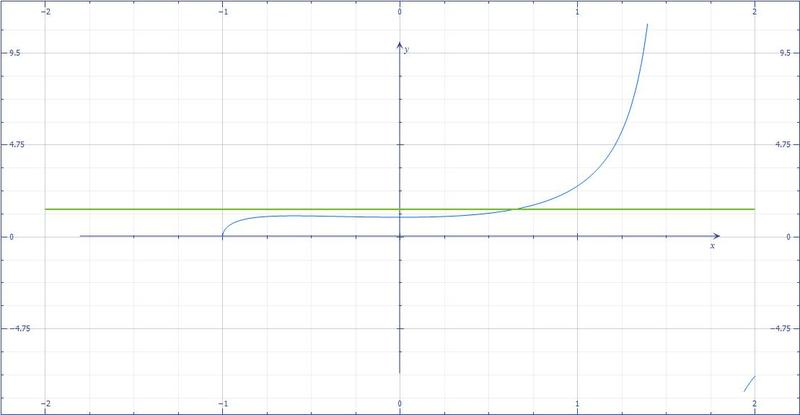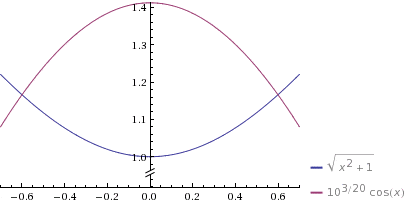# Isolating a function variable

Tyrone123

## Homework Statement

Im supposed to calculate the value of x for

20*log10($\frac{\sqrt{x^2 +1}}{cos(x)}$) = 3

## The Attempt at a Solution

I start off by dividing by 20 on both sides so I just have the expression with log.. and my idea henceforth is to take the inverse of cosine to get rid of cos(x), and square the square root to cancel out.. but the logarithm part has me stuck.. and im not sure how to get rid of/move it.. ive thought about multiplying with its inverse, but that has not gotten me any results.. any tips?

Last edited:

## Answers and Replies

Robert1986
How about $e$?

But I can't really tell what to do after that.

Staff Emeritus
Homework Helper
Gold Member

## Homework Statement

I'm supposed to calculate the value of x for

20*log$\frac{\sqrt{x^2 +1}}{\cos(x)}$

## The Attempt at a Solution

I start off by dividing by 20 on both sides so I just have the expression with log.. and my idea henceforth is to take the inverse of cosine to get rid of cos(x), and square the square root to cancel out.. but the logarithm part has me stuck.. and I'm not sure how to get rid of/move it.. I've thought about multiplying with its inverse, but that has not gotten me any results.. any tips?

## The Attempt at a Solution

You edited your original post!

Now, there is no equation!

(Formerly it said 20*log$\frac{\sqrt{x^2 +1}}{\cos(x)}=3\ .$)

Tyrone123
You edited your original post!

Now, there is no equation!

(Formerly it said 20*log$\frac{\sqrt{x^2 +1}}{\cos(x)}=3\ .$)

woops, I edited log to log10.. and suddenly the equation was gone :b

ohms law
I think propertieds of logs are important here.

log10 u/v = log10(u)-log10(v)

So, your original equation can be rewritten as:
20(log10(u)-log10(v))=3
with the numerator and denominator substituted for u and v, respectively. (hopefuly that makes sense)

Does that help?

Tyrone123
I think propertieds of logs are important here.

log10 u/v = log10(u)-log10(v)

So, your original equation can be rewritten as:
20(log10(u)-log10(v))=3
with the numerator and denominator substituted for u and v, respectively. (hopefuly that makes sense)

Does that help?

Possibly, ill try

Tyrone123
Not really.. I end up with a slightly prettier equation, but im not sure how to isolate x from log(cos(x)

ohms law
ah, well, there's a trig identity to help with that, I'm sure...
Off of the top of my head, there's always cos^2(x)+sin^2(x)=1, so...
I'll have to look some stuff up and see if it'll help.

Staff Emeritus
Homework Helper
Gold Member
If $\log_{10}(A)=B\,,$ then $A=10^B\ .$

Robert1986
All I see right now is something like this (where I'm using 10 instead of e) $\sqrt{x^2 + 1} = a \cos{x}$ where $a = 10^{3/20}$ then use the trig identity ohms law mentioned. (But multiply the identity by -1)

Tyrone123
ah, well, there's a trig identity to help with that, I'm sure...
Off of the top of my head, there's always cos^2(x)+sin^2(x)=1, so...
I'll have to look some stuff up and see if it'll help.

Hmm.. so far I have at least gotten rid of the log part, and I now have:

$\frac{\sqrt{x^2 +1}}{cos(x)}$ = 10$\frac{3}{20}$

Which I can rearrange a bit.. but that cos(x) is causing me troubles!

ohms law
fyi, for the numerator portion, I simplified it to 1/3 log(x)

Staff Emeritus
Homework Helper
Gold Member
Hmm.. so far I have at least gotten rid of the log part, and I now have:

$\frac{\sqrt{x^2 +1}}{\cos(x)}$ = 10$\frac{3}{20}$

Which I can rearrange a bit.. but that cos(x) is causing me troubles!

I do believe that solving this requires using a numerical method.

ohms law
1/cos(x) would = sec(x)...
so, log(1/cos(x)) = log(1) - log(sec(x))
since log(1) = 0, we can just get rid of that

just not sure how much that helps

ohms law
I do believe that solving this requires using a numerical method.

so, you're saying just plug and puke?
:)

Staff Emeritus
Homework Helper
Gold Member
so, you're saying just plug and puke?
:)
I've reviewed my posts, and no, I didn't say that. (LOL !)

OP may want to put his equation into a more suitable form before trying a numerical method.

Also, a graph may help in choosing a starting approximation.

Last edited:
ohms law
Hmm.. so far I have at least gotten rid of the log part, and I now have:

$\frac{\sqrt{x^2 +1}}{cos(x)}$ = 10$\frac{3}{20}$

Which I can rearrange a bit.. but that cos(x) is causing me troubles!

I missed this earlier, but... I'm not sure what you mean with that equality. It's not true as stated (see graph):ohms law
I do believe that solving this requires using a numerical method.

I guess that I'm just not clear what you mean by "requires using a numerical method".
I feel like I should know what you mean, but...Tyrone123
I missed this earlier, but... I'm not sure what you mean with that equality. It's not true as stated (see graph):
View attachment 51351

I dont mean anything in particular, just me trying to isolate x in the equation

Staff Emeritus
Homework Helper
Gold Member
Hmm.. so far I have at least gotten rid of the log part, and I now have:

$\frac{\sqrt{x^2 +1}}{cos(x)}$ = 10$\frac{3}{20}$

Which I can rearrange a bit.. but that cos(x) is causing me troubles!

I missed this earlier, but... I'm not sure what you mean with that equality. It's not true as stated (see graph):
View attachment 51351
Actually, that equality is true !

It leads to $\displaystyle \sqrt{x^2+1}=10^{3/20}\cos(x)\ .$

Tyrone123,
Notice that $\left(10^{3/20}\right)^2\approx1.99526\,,$ which is very nearly 2, so that $10^{3/20}$ is just a little less that $\sqrt{2}\ .$

Also, it may help to square that above equation, which then becomes:
$\displaystyle x^2 +1=10^{3/10}\cos^2(x)\ .$​

For small values of x, a good approximation for cos(x) is:
$\displaystyle \cos(x)\approx 1-\frac{x^2}{2}\ .$​

Plug that into the previous equation and solve for x to get a good starting place for any numerical method you choose.

ohms law
Actually, that equality is true !

It leads to $\displaystyle \sqrt{x^2+1}=10^{3/20}\cos(x)\ .$

man, trig makes my head hurt... :grumpy:
I don't get it. I'd drop it, but my sense is that this is part of the problem that Tyrone is having too (maybe I'm wrong, but...).

I know that graphs don't prove anything, but the graphs are (extremely) different (even with the cos(x) product added to the one side), so... I'm confused.

Staff Emeritus
Homework Helper
Gold Member
Here are the graphs of $y=\sqrt{x^2+1}$ and $y=10^{3/20}\cos(x)$ overlaid on the same graph (from WolframAlpha).#### Attachments

Staff Emeritus
Homework Helper
Gold Member
I missed this earlier, but... I'm not sure what you mean with that equality. It's not true as stated (see graph):
View attachment 51351
What is graphed here?Staff Emeritus
Homework Helper
Gold Member
man, trig makes my head hurt... :grumpy:
I don't get it. I'd drop it, but my sense is that this is part of the problem that Tyrone is having too (maybe I'm wrong, but...).

I know that graphs don't prove anything, but the graphs are (extremely) different (even with the cos(x) product added to the one side), so... I'm confused.
Take Tyrone's original equation.
$\displaystyle 20\,\log_{10}\left(\frac{\sqrt{x^2 +1}}{\cos(x)}\right)=3\$​

Do a little basic algebra & apply some of the log rules you suggested:
$\displaystyle \log(\sqrt{x^2+1})-\log(\cos(x))=\frac{3}{20}$

$\displaystyle \log(\sqrt{x^2+1})=\frac{3}{20}+\log(\cos(x))$

If you use each side as the exponent with a base of 10, you get:
$\displaystyle \sqrt{x^2+1}=10^{3/20}\cos(x)\$​